# How to find the number of Wednesdays in a year in Excel

So in this tutorial we’ll learn how to find the number of times a particular day i.e. Wednesday will occur between two dates by following the steps mentioned below. As there is no direct formula to achieve the goal so, we’ll use a workaround approach.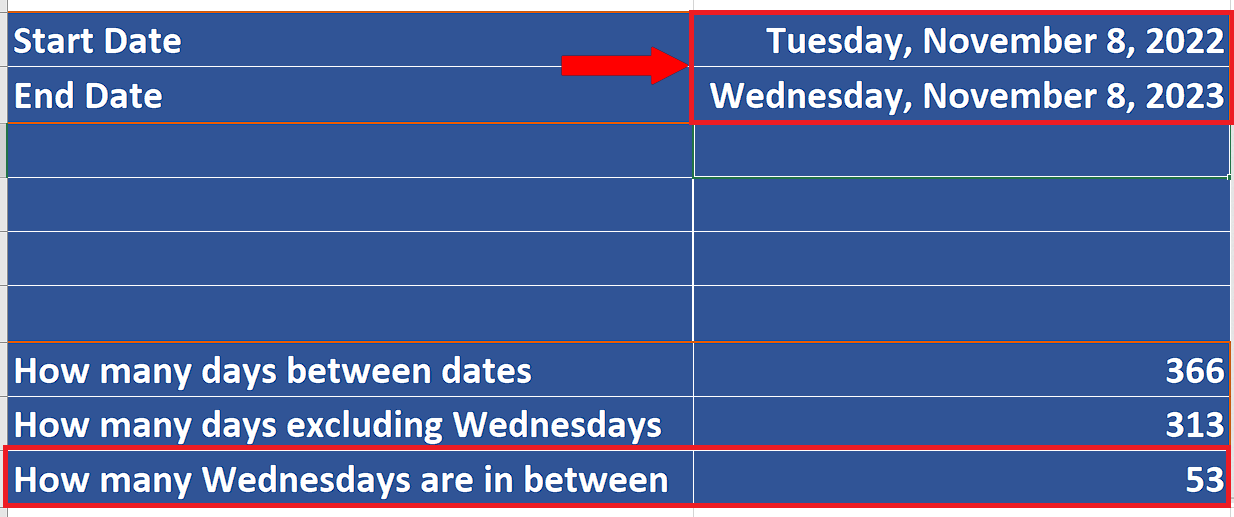While working with dates in Excel, there is often a requirement to find the number of dates or days in between two dates. Excel has some very useful formulas to work with dates and days but there is no specific formula to find out the number of a particular day occurring between two dates.

### Step 1 – Setup the start and end dates to create a date range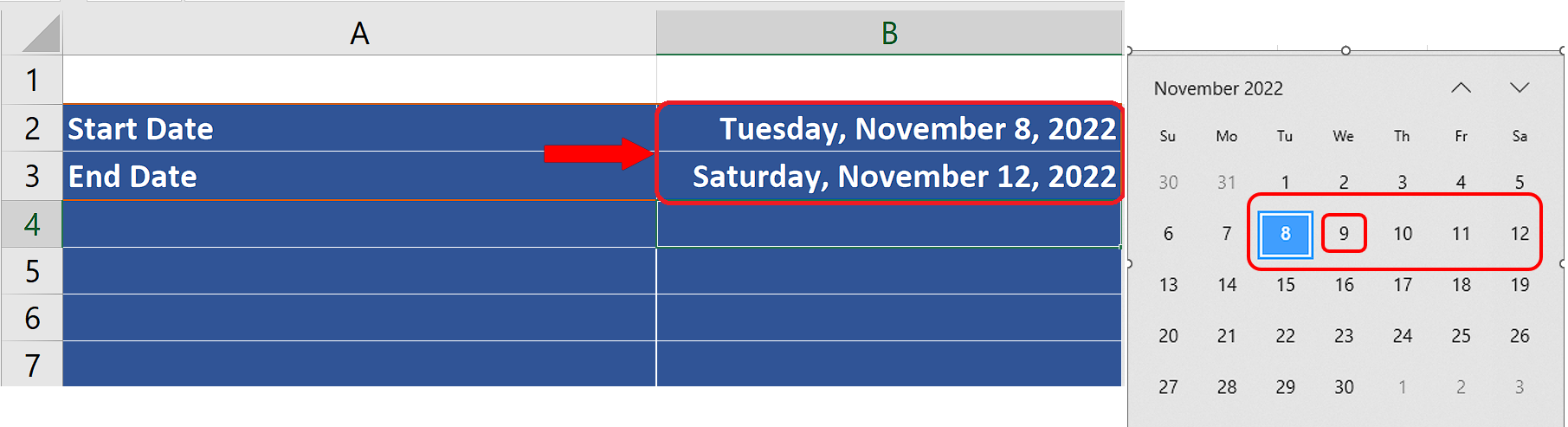– First setup two dates i.e. the start and end dates. We’ll first use a small date range and check the validity of the formula and then extend the formula to find the number of Wednesdays through one whole year.

– So we will take the dates from Nov 8, 2022 to Nov 12, 2022 so that we know there is only 1 Wednesday between the dates.

### Step 2 – Calculate the total number of days in between two dates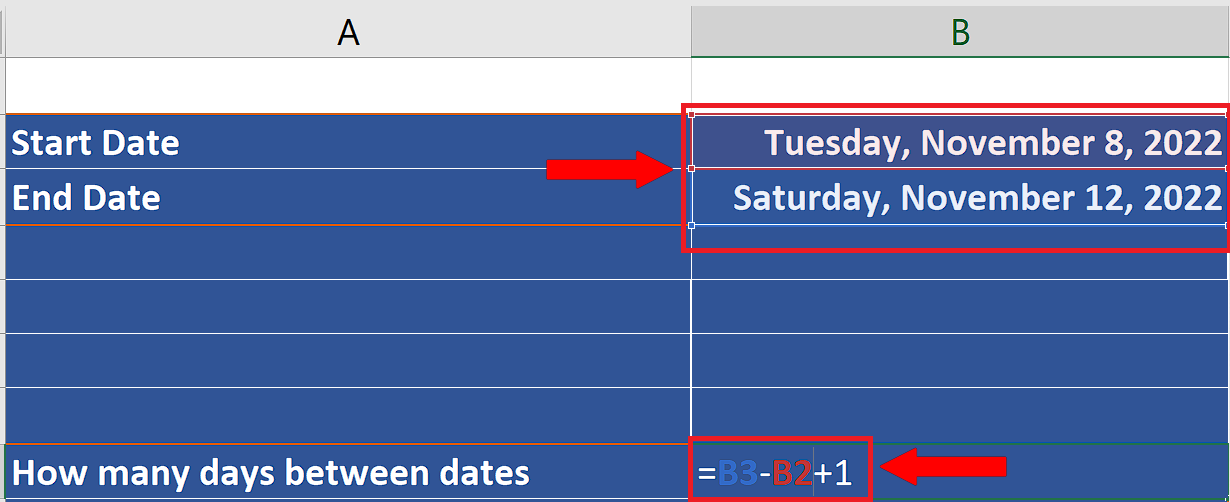– Now we’ll calculate the total number of days in between the start and end dates. If we find out the difference of the dates by simple subtraction then we’ll get the days in between and the start date or end date will not be included. So, to overcome this issue we’ll add 1 manually to the subtraction of the End and Start date.

### Step 3 – Calculate the total number of days in between two dates excluding Wednesday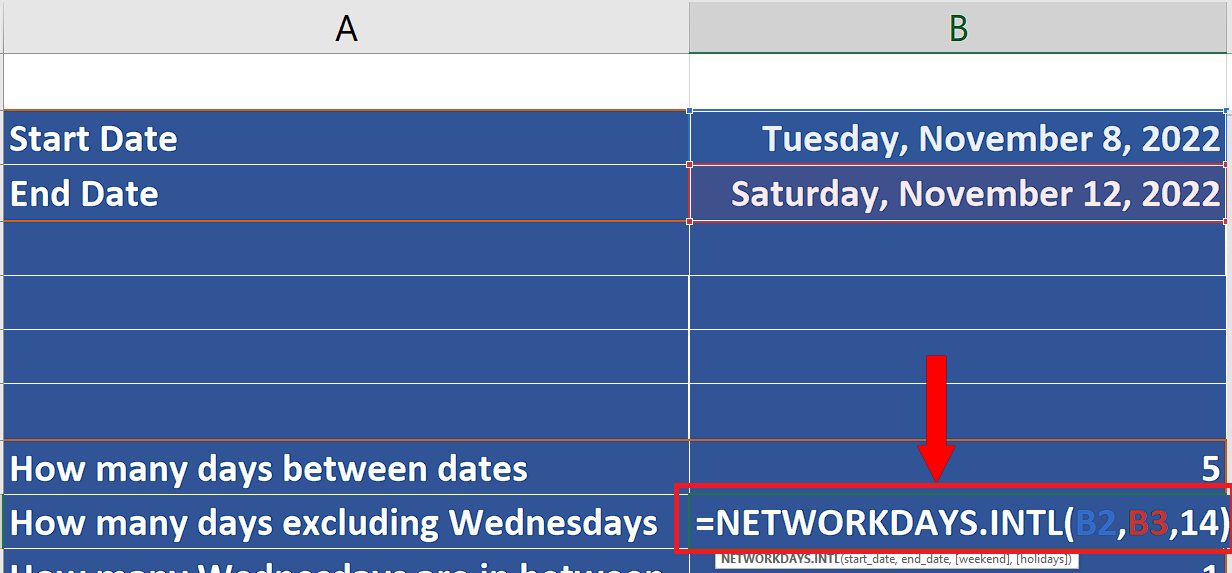– Now we’ll calculate the number of days in between the two dates excluding Wednesday. To do this we’ll use a simple formula i.e.
NETWORKDAYS.INTL(start_date, end_date, [weekend], [holidays])

This formula will calculate the net working days only by excluding the weekends.

The first two parameters of the formula are pretty straightforward which are start date and end dates i.e. B2 and B3 in our case.

By default the weekends are considered to be Saturday and Sunday. However, by using the correct option in the third parameter. It helps us to nominate any day as the weekend and then this day will be excluded from the calculation of the number of working days. So, we’ll choose 14 here which tells Excel that only Wednesday is the weekend.

– Implement the above formula in an appropriate cell as shown below;
=NETWORKDAYS.INTL(B2,B3,14)

### Step 4 – Calculate the number of Wednesdays between start and end date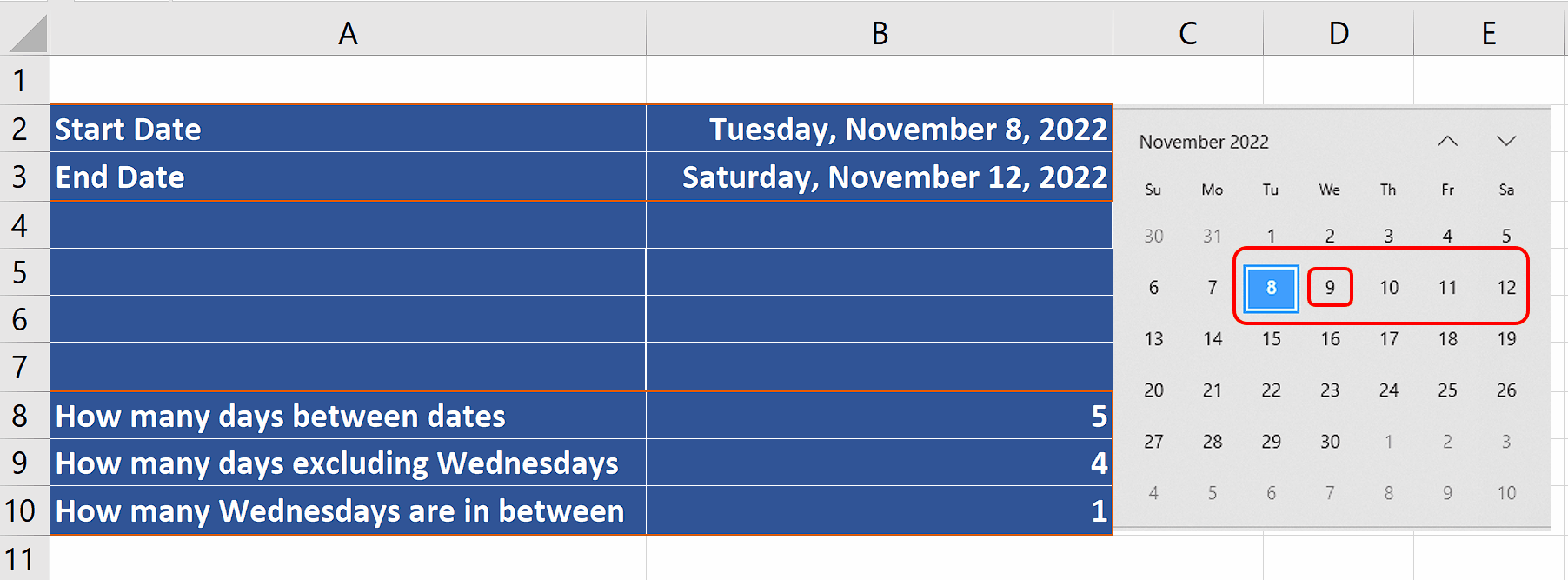– Now we’ll calculate the final thing i.e. number of Wednesdays by simply finding out the difference between the numbers calculated in step 2 and step 3 previously.

– This will give us the number of Wednesdays between start and end dates. In our current dataset we have chosen the dates from Nov 8, 2022 to Nov 12, 2022 and there is only 1 Wednesday between the dates. So we get 1 initially. However, we can extend the range to calculate the number of Wednesdays from Nov 8, 2022 to Dec 01, 2022 which are 4 as shown below.

### Step 5 – Calculate the number of Wednesdays in one year– The same formula can be used to find the number of Wednesdays in one year by choosing the start and end dates accordingly.

– Therefore, change the end date to Nov 8, 2023. The calculations will be automatically updated and you will get the number of Wednesdays in a year in the last row i.e. 53.